Study Guide

# Hyperbolas

## Hyperbolas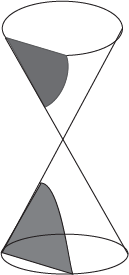Do not adjust your TV set. Those are not a pair of parabolas frolicking in that cone. No, they are actually a single conic section, and our last one, the hyperbola.

We wouldn't blame you for mistaking it for some parabolas, though. Hyperbolas get that a lot. Each half has a directrix outside of the curve, a vertex, and a focus that is inside the curve, just like a parabola. Hyperbolas also have an axis of symmetry, the transverse axis, that goes through all of the vertices, foci, and the center. Fancy shmancy. The big difference from parabolas is that both these curves are juggled at the same time.

Actually, the big HUGE difference is that hyperbolas have two asymptotes. They crisscross through the hyperbola's center, and the hyperbola's arms approach but never touch them as they all wander off into infinity.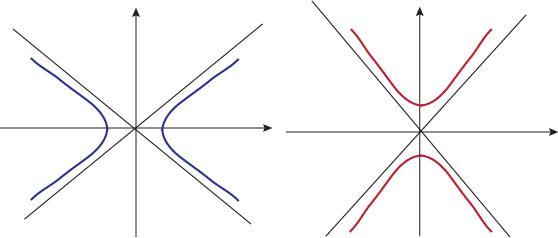As you probably could guess, hyperbolas can be horizontal or vertical. The transverse axis has the same orientation as the hyperbola, horizontal or vertical. Which way the graph opens all depends on whether x or y come first in the equation. But that's getting ahead of ourselves, and being both ahead and behind ourselves gets confusing quickly.

• ### Equation of a Hyperbola

What we really really want is zigazig ha, but we'll settle for the equation of a hyperbola. Like a hyperbola itself, though, we've got a twofer here.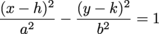This is the equation we use for horizontal hyperbolas—x is the positive term, and so the graph opens to the left and right.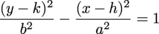The only difference for an up-and-down hyperbola is that now y is positive and x is negative. In either case, the transverse axis—and so the center, foci, vertices, and all their fanboys—follows the direction of the positive term, too.

Hyperbolas have a body like two parabolas, an equation like an ellipse, and the soul of a champion. Hyperbolas have a center at (h, k), which will be right in the middle of the two curves.

To find the vertices, shout out "Marco" and wait for them to say "Polo" back. Or check out the positive term in the equation. Use the square root of the denominator, just like we did with ellipses, to find the distance from the center to the vertices. If it's x, use a; if it's y, use b. If it's a rash, go see a doctor.

Hyperbolas only have the two vertices, so at first the second variable seems useless. Harsh but true. That's not actually the case, though. It helps us find the slopes of the asymptote lines.The slopes are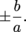. It doesn't matter if the hyperbola opens left and right, or up and down. These are the slopes for the two asymptotes. Both asymptotes meet at the center before passing off into parts unknown. We can use the point-slope form of a line to give the asymptotes' equations: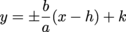The last thing we need to track down are the foci. We need to keep those mathematicians happy, and yes, this means we're skipping finding the directrixes again. We're sorry if you hate mathematicians or love the directrix.

Our equation for foci from the ellipse will not work here. Luckily we have an equation that does work, and we're just going to give it to you. Plus, it's even easier to remember. Hyperbolas, you're all right in our book. The equation for finding the distance, f, from the center to the foci is a2 + b2 = f2. It looks a little Pythagorean, doesn't it? Like with the ellipse before, it's actually more complicated than that, but we're just going to move on. We don't think you'll mind.

### Sample Problem

Find the center, vertices, foci, and asymptotes for this hyperbola: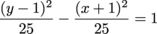We're starting off with a hyperbola with a positive y term. That should put us in an up-and-down kind of mood, so we'll remember that this is a vertical hyperbola. The transverse axis is going to be vertical, so the center, vertices, and foci will all share an x term and only vary by y.

When finding the center, we won't let the order of x and y trip us up. h always goes with x, even if x is second and being subtracted. The center is at (-1, 1).

Now we need a and b, pulled out from beneath x and y. Again, we don't care about the order of the two, and you can't make us. Taking the square root of both denominators, we get a = b = 5. Now to put them to work.

A hyperbola hinges on its vertices, and so we've got to rise to the challenge of finding them. Well, one of them. The other will be below the center. Remember, our parabola opens up and down, so our vertices and foci will be a up and a down from the center. That gives us vertices of (-1, 6) and (-1, -4).

Speaking of foci, why not find them now? Well, we'd rather go find a sunny spot on the beach to lie on, but we're kind of in the middle of something here. And not anywhere near a beach. Bummer. But at least we can find f. For a hyperbola:

f2 = a2 + b2

= 25 + 25 = 50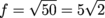This is the distance from the center to the foci. It is bigger than a, just like we would expect, because the foci hang out in the inner sanctum of the curve, past the vertices. They've got a great inner sanctum, with high-speed wifi, beanbag chairs galore, and a gigantic mini-fridge. Yes, gigantic and mini at the same time. To reach their sweet hangout spot, go to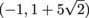or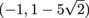.

Last but not least, we hunt down the asymptotes. They're easier to catch than wascally wabbits, at least. The slopes are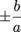. That's easy in this case, ±1. Now we'll just plug that and the center of the hyperbola into the point-slope form:

yy1 = m(xx1)

y – 1= ±1(x + 1)

y = ±(x + 1) + 1

Let's get rid of that ± sign and show each equation separately.

y = x + 2

y = -x

Equations: caught. Now we will show off everything we've found in a nice and neat graph.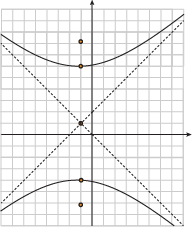The asymptotes make graphing a hyperbola easy. Just don't cross them and we'll be good.

### Sample Problem

Find the center, vertices, foci, and asymptotes for this hyperbola: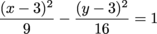This time, we have a horizontal hyperbola. x is our positive term, so it's going to open to the left and right. The center, vertices, and foci are all lying on their backs on the transverse axis.

The center of the hyperbola sits pretty at (3, 3). a and b are under x and y, and they equal 3 and 4. But which one will lead us to the vertices, and which one leads us to jack squat? Unlike ellipses, hyperbolas don't care which one is bigger; they just want the one with the positive term. That means our distance to the vertices is a = 3, so the vertices are (0, 3) and (6, 3).

Let's blast past those vertices with the foci. We'll use our not-actually-but-looks-like-it Pythagorean-ish equation to do it.

f2 = a2 + b2

= 9 + 16 = 25

f = 5

The foci keep on trucking along the horizontal transverse axis. That means we keep moving horizontally from the center, so the foci are at (-2, 3) and (8, 3).

All that's left now are the asymptotes. Remember that the equation for the asymptotes doesn't change between horizontal and vertical hyperbolas. They're set in their ways, and refuse to be anything other than.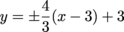So the asymptotes are: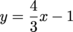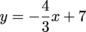The hyperbola can now be graphed. We think we'll do it, too, even though the question didn't ask for it.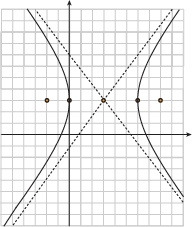Graphs're nice. Another problem to work on, not so much.

### Sample Problem

Find the equation of the vertical hyperbola that fits the following:

Center: (0, 0), a = 1, and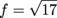O hyperbola, hyperbola, wherefore art thou hyperbola? Actually, we want to know where this hyperbola is, not why, so scratch that. We already know the center and a, so we're most of the way there already. We also have f, so we can already find b.

f2 = a2 + b2

17 = 1 + b2

b = 4

Problem over, right? Not quite. And hey, that kind of rhymes. A hyperbola has one positive and one negative term, so which one is which? Our clue is that it is a vertical hyperbola. Vertical means up and down, which means y is in charge here.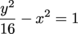And that, as they say, is that.